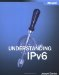IPv4 addresses are represented in dotted-decimal format. The 32-bit IPv4 address is divided along 8-bit boundaries. Each set of 8 bits is converted to its decimal equivalent and separated by periods. For IPv6, the 128-bit address is divided along 16-bit boundaries, and each 16-bit block is converted to a 4-digit hexadecimal number and separated by colons. The resulting representation is called colon hexadecimal.

The following is an IPv6 address in binary form:

` 0010000111011010000000001101001100000000000000000010111100111011 0000001010101010000000001111111111111110001010001001110001011010 `

The 128-bit address is divided along 16-bit boundaries:

` 0010000111011010  0000000011010011  0000000000000000  0010111100111011 0000001010101010  0000000011111111  1111111000101000  1001110001011010 `

Each 16-bit block is converted to hexadecimal and delimited with colons. The result is:

` 21DA:00D3:0000:2F3B:02AA:00FF:FE28:9C5A `

IPv6 address representation is further simplified by suppressing the leading zeros within each 16-bit block. However, each block must have at least a single digit. With leading zero suppression, the result is:

` 21DA:D3:0:2F3B:2AA:FF:FE28:9C5A `

Number System Choice for IPv6

Hexadecimal (the Base16 numbering system), rather than decimal (the Base10 numbering system), is used for IPv6 because it is easier to convert between hexadecimal and binary than it is to convert between decimal and binary. Each hexadecimal digit represents four binary digits.

With IPv4, decimal is used to make the IPv4 addresses more palatable for humans and a 32-bit address becomes 4 decimal numbers separated by the period (.) character. With IPv6, dotted decimal representation would result in 16 decimal numbers separated by the period (.) character. IPv6 addresses are so large that there is no attempt to make them palatable to most humans, with the exception of some types of IPv6 addresses that contain embedded IPv4 addresses. Configuration of typical end systems is automated and end users will almost always use names rather than IPv6 addresses. Therefore, the addresses are expressed in a way to make them more palatable to computers and IPv6 network administrators who understand the semantics and relationship of hexadecimal and binary numbers.

Table 3-2 lists the conversion between binary, hexadecimal, and decimal numbers.

Table 3-2. Converting Between Binary, Hexadecimal, and Decimal Numbers

0000

0

0

0001

1

1

0010

2

2

0011

3

3

0100

4

4

0101

5

5

0110

6

6

0111

7

7

1000

8

8

1001

9

9

1010

A

10

1011

B

11

1100

C

12

1101

D

13

1110

E

14

1111

F

15

## Compressing Zeros

Some types of IPv6 addresses contain long sequences of zeros. To further simplify the representation of IPv6 addresses, a single contiguous sequence of 16-bit blocks set to 0 in the colon hexadecimal format can be compressed to ::, known as a double colon.

For example, the link-local address of FE80:0:0:0:2AA:FF:FE9A:4CA2 can be compressed to FE80::2AA:FF:FE9A:4CA2. The multicast address FF02:0:0:0:0:0:0:2 can be compressed to FF02::2.

You cannot use zero compression to include part of a 16-bit block. For example, you cannot express FF02:30:0:0:0:0:0:5 as FF02:3::5, but FF02:30::5 is correct.

How Many Bits in ::?

To determine how many 0 bits are represented by the ::, you can count the number of blocks in the compressed address, subtract this number from 8, and then multiply the result by 16. For example, in the address FF02::2, there are two blocks (the "FF02" block and the "2" block.) The number of bits expressed by the :: is 96 (96 = (8 - 2) x 16). Zero compression can be used only once in a given address. Otherwise, you could not determine the number of 0 bits represented by each instance of ::.

## IPv6 Prefixes

The prefix is the part of the address where the bits have fixed values or are the bits of a route or subnet identifier. Prefixes for IPv6 subnet identifiers and routes are expressed in the same way as Classless Inter-Domain Routing (CIDR) notation for IPv4. An IPv6 prefix is written in address/prefix-length notation.

For example, 21DA:D3::/48 is a route prefix and 21DA:D3:0:2F3B::/64 is a subnet prefix. As described earlier in this chapter, the 64-bit prefix is used for individual subnets to which nodes are attached. All subnets have a 64-bit prefix. Any prefix that is less than 64 bits is a route or address range that is summarizing a portion of the IPv6 address space.

IPv4 implementations commonly use a dotted decimal representation of the network prefix known as the subnet mask. A subnet mask is not used for IPv6. Only the prefix length notation is supported.

An IPv6 prefix is relevant only for routes or address ranges, not for individual unicast addresses. In IPv4, it is common to express an IPv4 address with its prefix length. For example, 192.168.29.7/24 (equivalent to 192.168.29.7 with the subnet mask 255.255.255.0) denotes the IPv4 address 192.168.29.7 with a 24-bit subnet mask. Because IPv4 addresses are no longer class-based, you cannot assume the class-based subnet mask based on the value of the leading octet. The prefix length is included so that you can determine which bits identify the subnet and which bits identify the host on the subnet. Because the number of bits used to identify the subnet in IPv4 is variable, the prefix length is needed to separate the subnet ID from the host ID.

In IPv6, however, there is no notion of a variable length subnet identifier. At the individual IPv6 subnet level for currently defined unicast IPv6 addresses, the number of bits used to identify the subnet is always 64 and the number of bits used to identify the host on the subnet is always 64. Therefore, while unicast IPv6 addresses written with their prefix lengths are permitted in RFC 2373, in practice their prefix lengths are always 64 and therefore do not need to be expressed. For example, there is no need to express the IPv6 unicast address FEC0::2AC4: 2AA:FF:FE9A:82D4 as FEC0::2AC4:2AA:FF:FE9A:82D4/64. Due to the 50-50 split of subnet and interface identifiers, the unicast IPv6 address FEC0::2AC4:2AA: FF:FE9A:82D4 implies that the subnet identifier is FEC0:0:0:2AC4::/64.Understanding Ipv6
ISBN: 0735612455
EAN: 2147483647
Year: 2005
Pages: 124
Authors: Joseph Davies

Similar book on Amazon